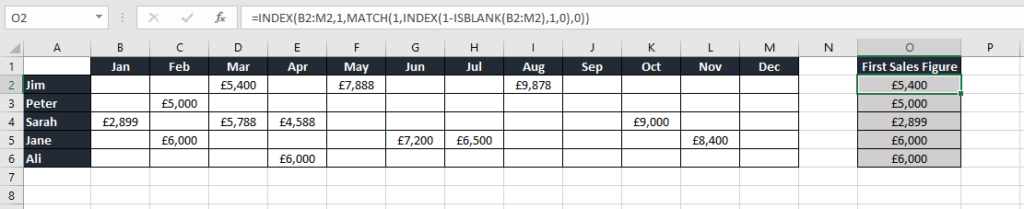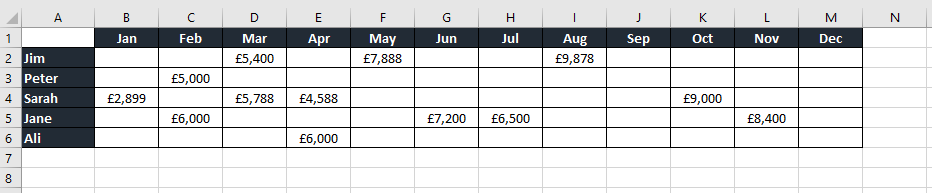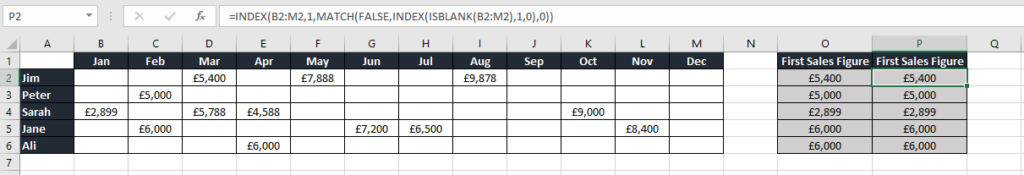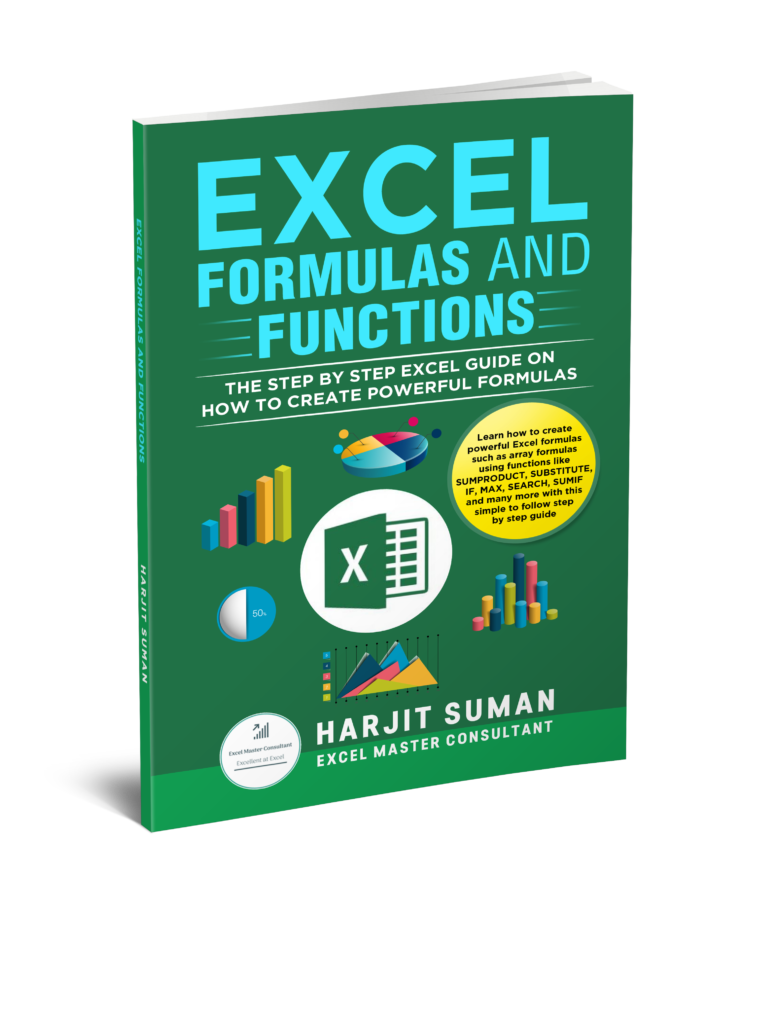# How to Find the First Non Blank Cell in a Row

Once you become proficient in Excel you can start to do some awesome things! In this post you will learn how to find the first non blank cell in a row. The formula used is a complex one. It combines the INDEX, MATCH and ISBLANK Excel functions. As a result, I will break the formula down so you can understand how each section of the formula works.

Now lets see how we can tackle the challenge below.

## How to Find the First Non Blank Cell in a Row of SalesA list of Sales people with their sales in months

### Challenge

Here we have a list of Sales people with their sales in different months. The challenge is to extract the first sales figure for each Sales person. For example, Jim’s first sale is in March. Peter’s first sale is in February, Sarah’s is in January and so on.

### SolutionFormula to extract the first sales figure for each Sales person

The formula in cell O2 is:

=INDEX(B2:M2,1,MATCH(1,INDEX(1-ISBLANK(B2:M2),1,0),0))

Even though the formula contains an array of cells it still results in a single value answer. You do not need to press Control + Shift + Enter when you enter the formula.

### How the Formula Works

So how does this formula work? Let’s break this formula down to understand how it works.

1. The ISBLANK function will return TRUE if a cell is blank and FALSE if the cell is non blank. Range B2:M2 will therefore return {TRUE, TRUE, FALSE, TRUE, FALSE, TRUE, TRUE, FALSE, TRUE, TRUE, TRUE, TRUE}
1. The formula will therefore read:

=INDEX(B2:M2,1,MATCH(1,INDEX(1-{TRUE,TRUE,FALSE,TRUE,FALSE,TRUE,TRUE,FALSE,TRUE,TRUE,TRUE,TRUE}),1,0),0))

1. The TRUE and FALSE values are treated as a 1 or a 0 in an array formula. This is achieved by specifying 1-ISBLANK(B2:M2). This converts the TRUE and FALSE to 1’s and 0’s. TRUE=1 and FALSE=0
1. The 1-ISBLANK(B2:M2) will therefore return a 0 for a blank cell and a 1 for a non blank cell. For example, B2 will return a 0 because 1-1 is 0. Cell D2 will return a 1 because 1-0 =1. Range B2:M2 will therefore return {0,0,1,0,1,0,0,1,0,0,0,0}. The formula will read:

=INDEX(B2:M2,1,MATCH(1,INDEX({0,0,1,0,1,0,0,1,0,0,0,0},1,0),0))

1. Normally the INDEX function returns a single value. However, if you enter a 0 in the column argument it will return an array of values. The result is {0,0,1,0,1,0,0,1,0,0,0,0}
1. MATCH(1,INDEX(1-ISBLANK(B2:M2),1,0),0) returns the first position of 1 in the array B2:M2. In the range B2:M2 the first position of 1 is in position 3, which is March. If you break this down it will look like this:

MATCH(1, {0,0,1,0,1,0,0,1,0,0,0,0},0)

1. You know that the third column contains the first non blank cell. You now need to return the value in this third column. This is where you use the INDEX function. To break this down the formula will be:

=INDEX(B2:M2,1,3)

1. The result is £5,400

## Additional Notes on How to Find the First Non Blank Cell in a Row

One point to note is that if all the cells are blank it will return a #N/A error.

There is an alternative formula which looks for FALSE as the first argument of the MATCH function instead of subtracting the ISBLANK function from 1.An alternative formula to find the first non blank cell in a row in cell P2

The formula is:

=INDEX(B2:M2,1,MATCH(FALSE,INDEX(ISBLANK(B2:M2),1,0),0))

You have now discovered you can create powerful array formulas using the ISBLANK, INDEX and MATCH Excel functions. I hope you enjoyed this tutorial on how to find the first non blank cell in a row. I would love to hear from my readers. If you have any comments or questions then please leave me a message below.

## Learn how to Create More Powerful Formulas

I hope this Excel tutorial has given you a taster to learn how to create even more powerful formulas. If so, then you can purchase my book Excel Formulas and Functions: The Step by Step Excel Guide on how to Create Powerful Formulas. This book will teach you how to write powerful array formulas. You will learn how to use advanced Excel functions such as SUMPRODUCT, SUBSTITUTE, REPT, SEARCH, FIND and many more.Excel Formulas and Functions: The Step by Step Excel Guide on how to Create Powerful Formulas

Here are some reviews from people who have purchased this book.

To learn more about this book and to purchase it from Amazon, then simply click one of the buttons below. You can also purchase this book in eBook format by visiting my shop.1.